# Exploratory analysis

Data visualization, part 1. Code for Quiz 7.

1. Load the R package we will use.
``````library(tidyverse)
``````
1. Quiz questions
• Replace all the ???s. These are answers on your moodle quiz.

• Run all the individual code chunks to make sure the answers in this file correspond with your quiz answers

• After you check all your code chunks run then you can knit it. It won’t knit until ??? are replaced

• The quiz assumes you have watched the videos had worked through the exercises in exercises_slides-1-49.Rmd

1. Pick one of your plots to save as your preview plot. Use the ggsave command at the end of the chunk of the plot that you want to preview.

# Question: modify slide 34

• Create a plot with the faithful dataset

• assign the variable waiting to the y-axis -color the points according to whether waiting is smaller or greater than 76

``````ggplot(faithful) +
geom_point(aes(x = eruptions, y = waiting,
colour = waiting > 77))
``````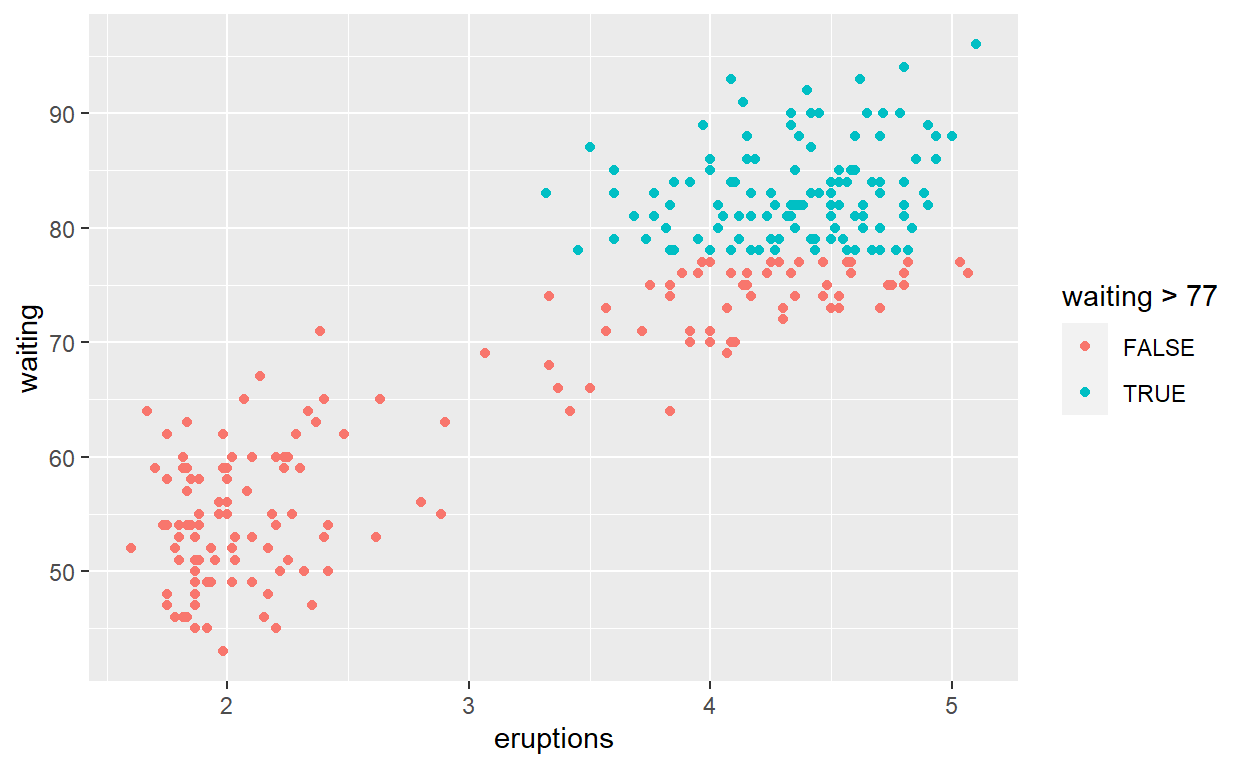# Question: modify intro-slide 35

• Create a plot with the faithful dataset

• assign the variable eruptions to the x-axis

• assign the variable waiting to the y-axis

• assign the color purple to all the points

``````ggplot(faithful) +
geom_point(aes(x = eruptions, y = waiting),
colour = "purple")
``````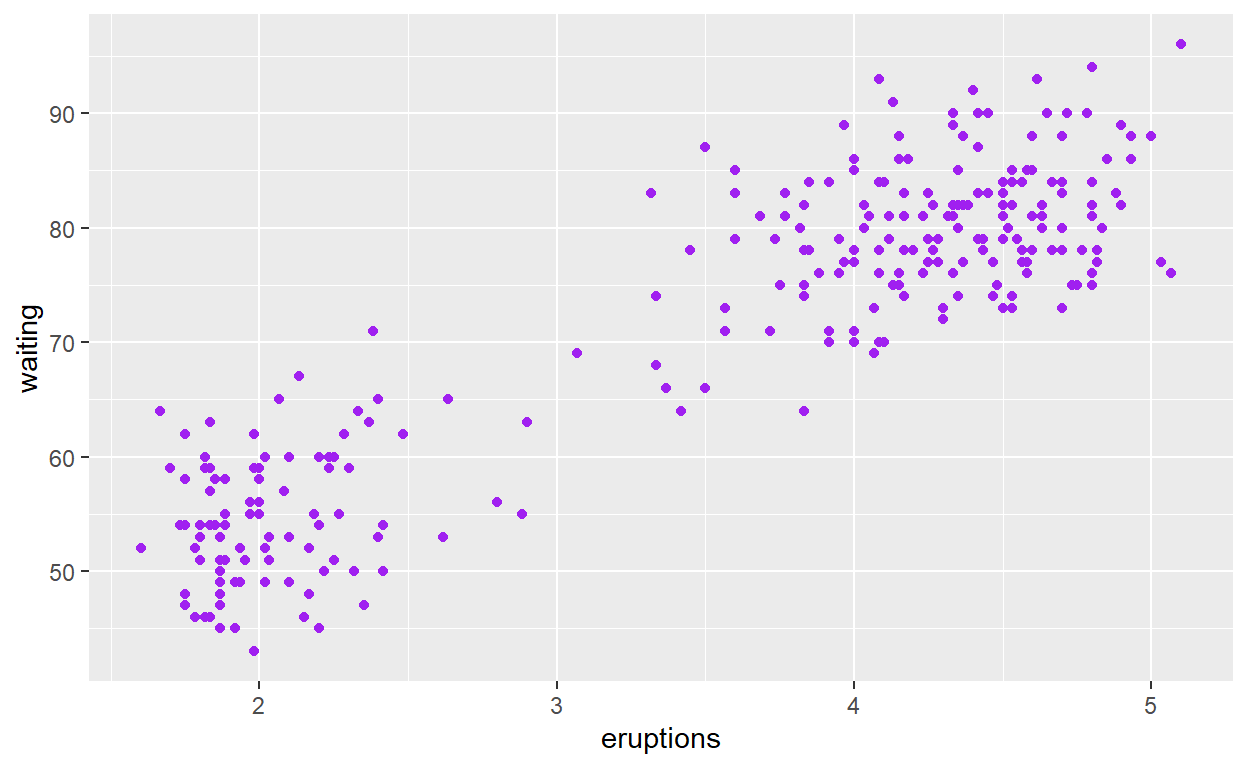# Question: modify intro-slide 36

• Create a plot with the faithful dataset

• use geom_histogram() to plot the distribution of waiting time

• assign the variable waiting to the x-axis

``````ggplot(faithful) +
geom_histogram(aes(x = waiting))
``````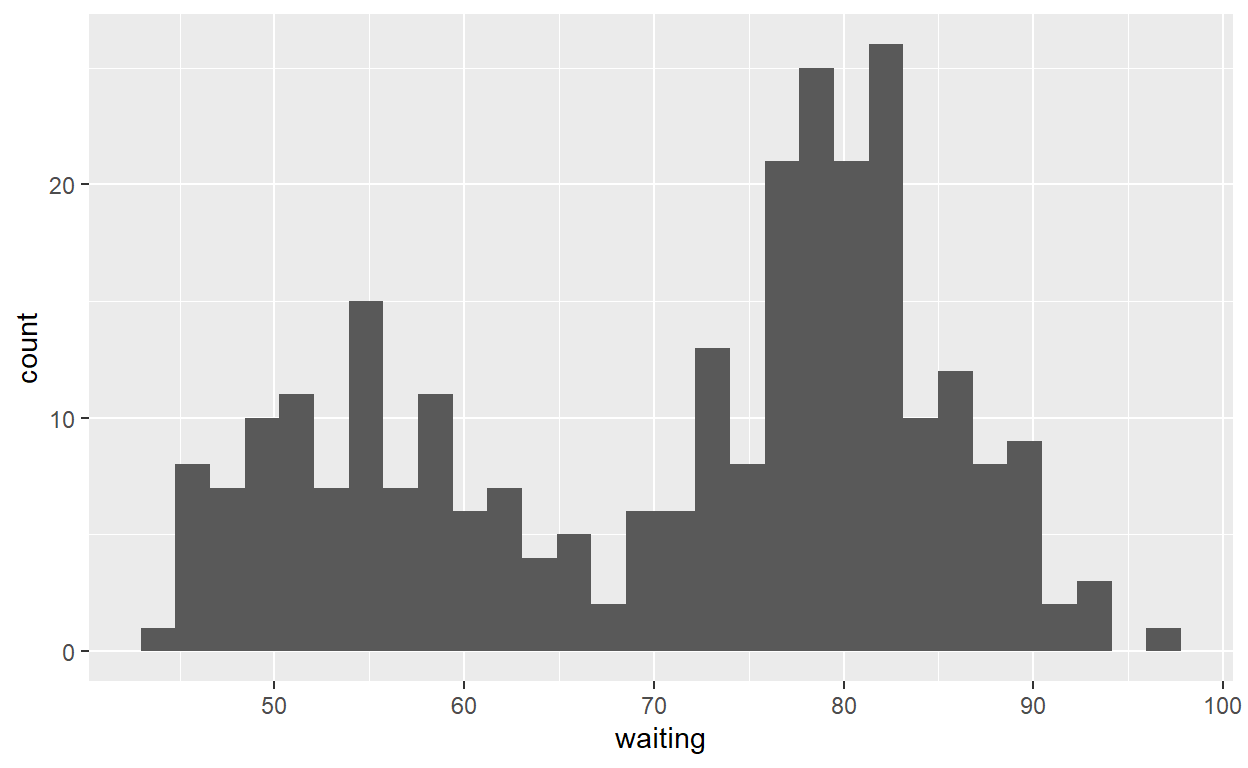# Question: modify geom-ex-1

• See how shapes and sizes of points can be specified here: https://ggplot2.tidyverse.org/articles/ggplot2-specs.html#sec:shape-spec

• Create a plot with the faithful dataeset

• assign the variable eruptions to the x-axis

• assign the variable waiting to the y-axis

• set the shape of the points to diamond

• set the point size to 5

• set the point transparency 0.9

``````ggplot(faithful) +
geom_point(aes(x = eruptions, y = waiting),
shape = "diamond", size = 5, alpha = 0.9)
``````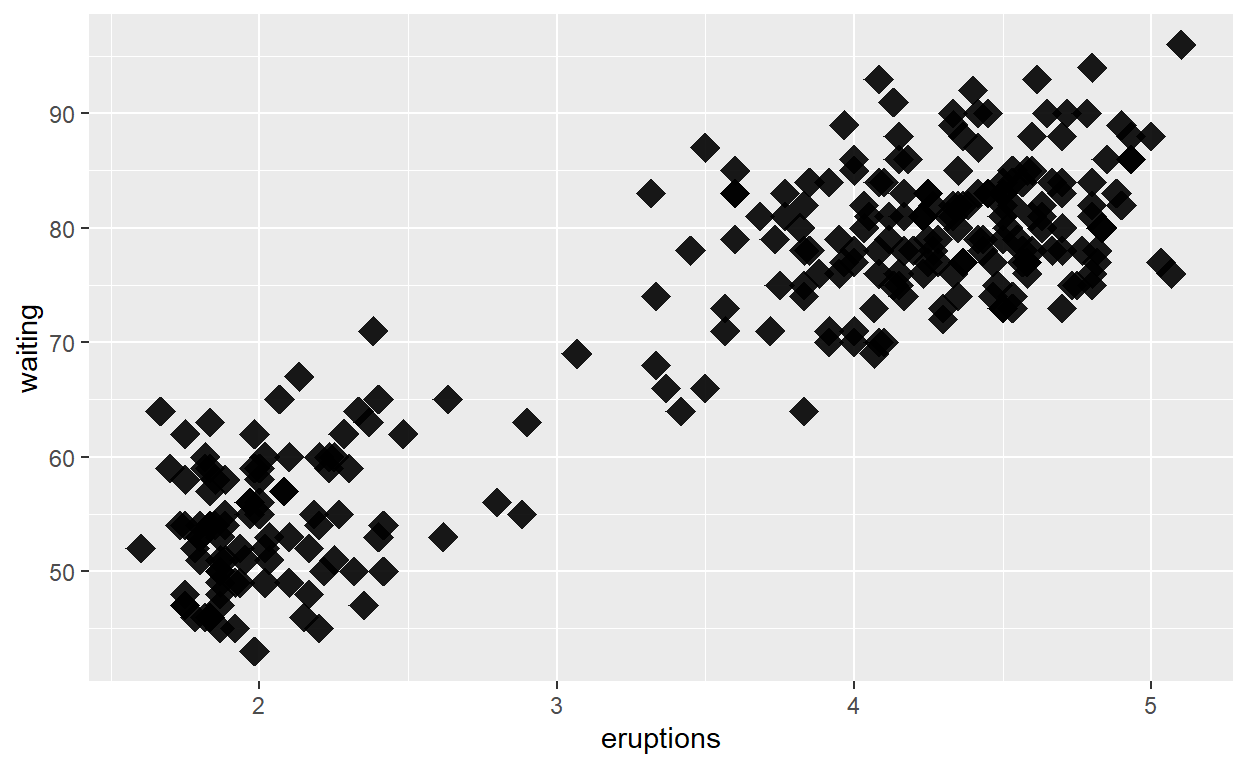# Question: modify geom_ex 2

• Create a plot with the faithful dataset

• use geom_histogram() to plot the distribution of the eruptions (time)

• fill in the histogram based on whether eruptions are greater than or less than 3.2 minutes

``````ggplot(faithful) +
geom_histogram(aes(x = eruptions, fill = eruptions > 3.2))
``````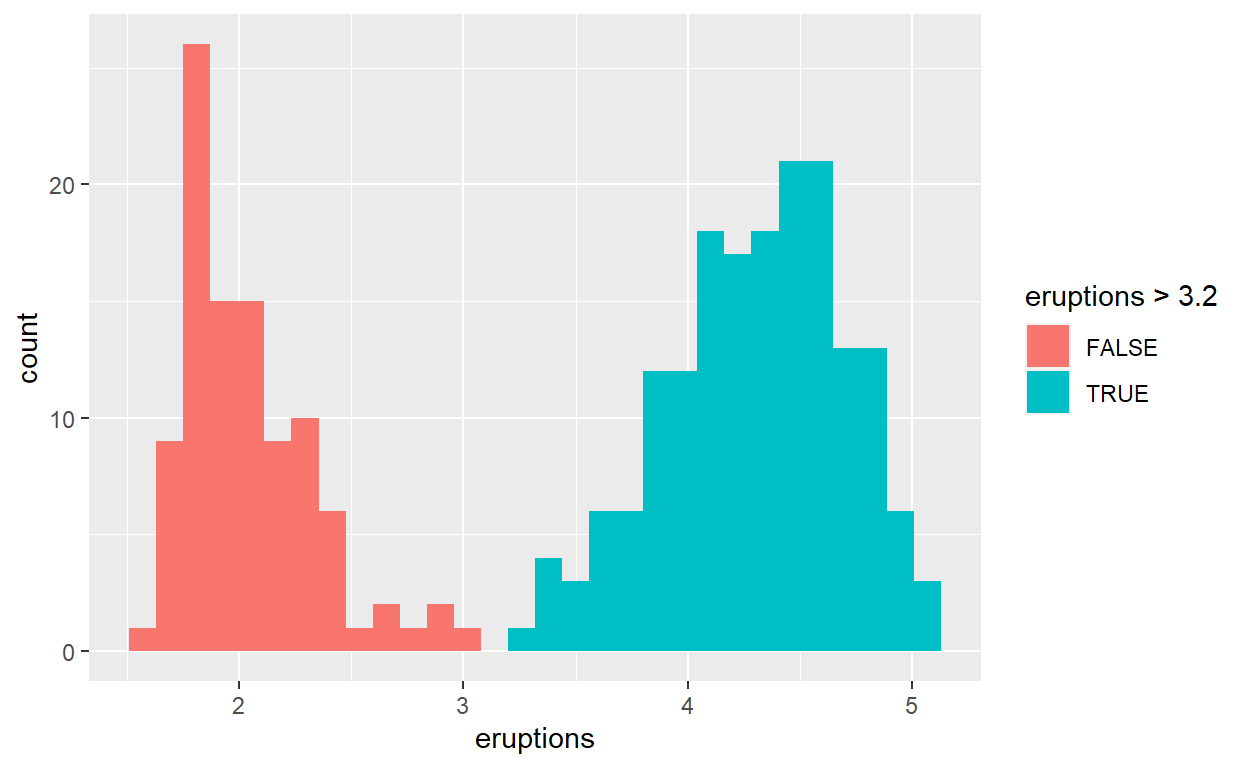# Question: modify stat-slide-40

• Create a plot with the mpg dataset

• add geom_bar() to create a bar chart of the variable manufacturer

``````ggplot(mpg) +
geom_bar(aes(x = manufacturer))
``````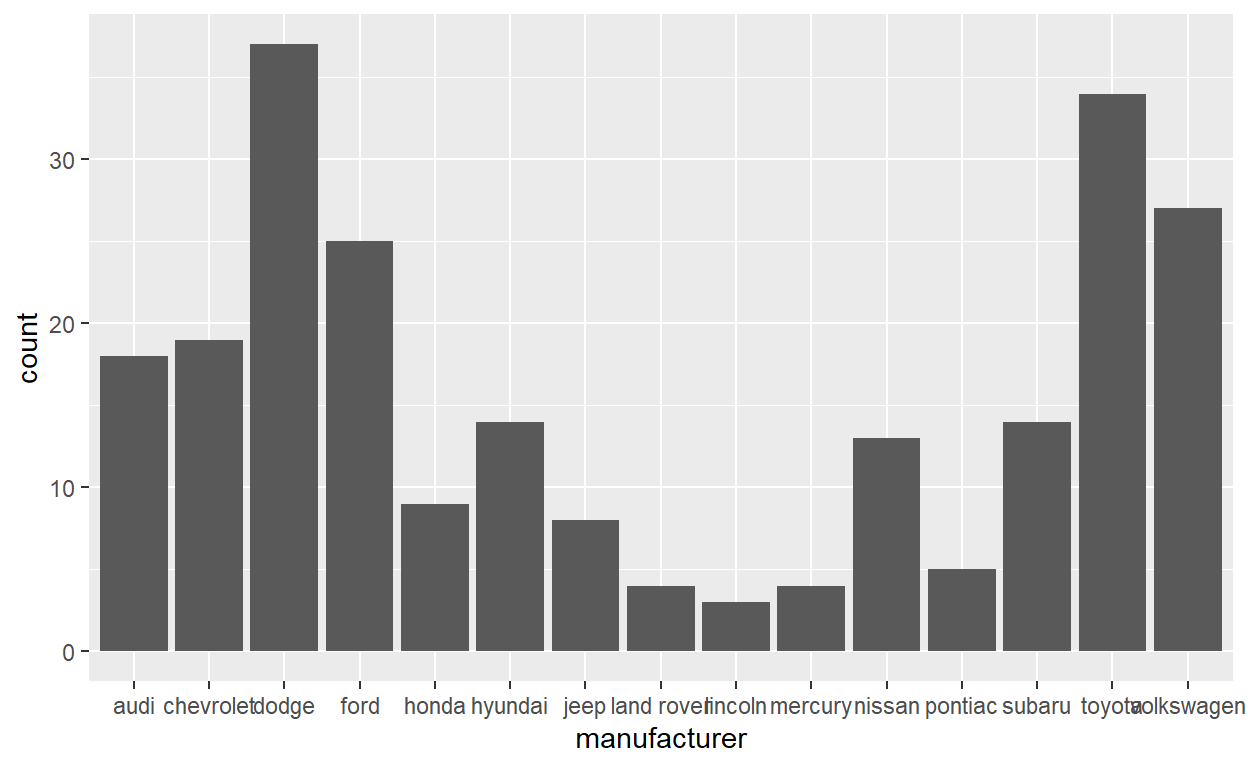# Question: modify stat-slide-41

• change code to count and to plot the variable manufacturer instead of class
``````mpg_counted <- mpg %>%
count(manufacturer, name = 'count')
ggplot(mpg_counted) +
geom_bar(aes(x = manufacturer, y = count), stat = 'identity')
``````# Question: modify stat-slide-43

• change code to plot bar chart of each manufacturer as a percent of total

• change class to manufacturer

``````ggplot(mpg) +
geom_bar(aes(x = manufacturer, y = after_stat(100 * count / sum(count))))
``````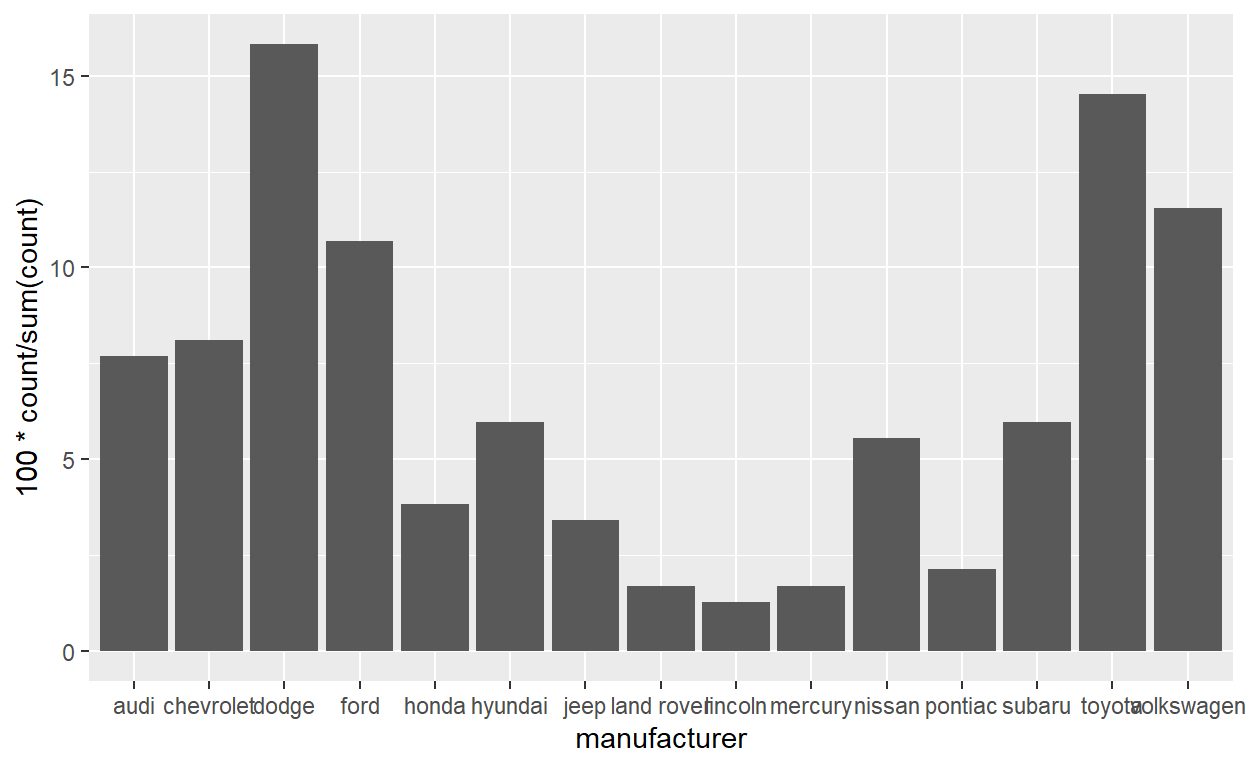# Question: modify answer to stat-ex-2

• use stat_summary() to add a dot at the median of each group

• color the dot blueviolet

• make the shape of the dot cross

• make the dot size 9

``````ggplot(mpg) +
geom_jitter(aes(x = class, y = hwy), width = 0.2) +
stat_summary(aes(x = class, y = hwy), geom = "point",
fun = "median", color = "blueviolet",
shape = "cross", size = 9)
``````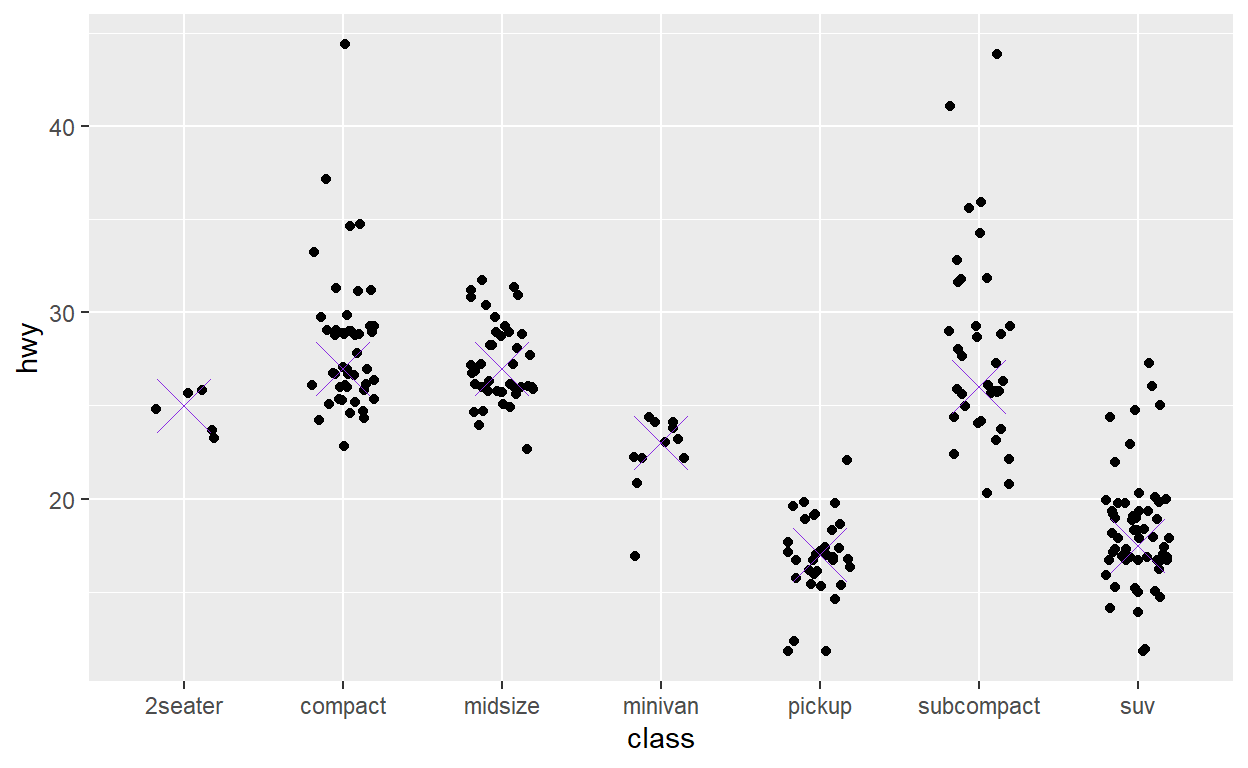``````ggsave(filename = "preview.png",
path = here::here("_posts", "2022-03-18-exploratory-analysis"))
``````